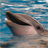# Power factor calculation for metering application

cancel
Showing results for
Did you mean:

## Power factor calculation for metering application

289 ViewsContributor I

Hi,

I have been using the metering library on KM14Z board. Currently I am facing problem in calculating the power factor using the Filter based algorithm of metering library. There are no API's available to calculate the power factor, therefore I am calculating it by dividing Active power with S(VA).

PF = P(Watts)/S(VA).

This calculation gives me wrong results. Could someone guide me on this issue.

Labels (1)
• ### Kinetis M Series MCUs

151 ViewsNXP TechSupport

Hi, Bharath,

In my opinion, the power factor is an angle, so it should be arccos(P/sqrt(P**2+Q**2)),P is active energy, Q is reactive energy, do you use the formula to compute it?

BR

Xiangjun rong